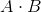Test: Linear Algebra

 1 Your friend Hector wants to multiply two matricesandas follows:. Unfortunately, Hector knows nothing about matrix dimensions. Which of the following statements will help Hector figure out whether it is possible for him to multiply?

The number of rows in matrixmust be equal to the number of columns in matrix.

The number of rows in matrixmust be equal to the number of rows in matrix.andmust both be square matrices, otherwise you cannot multiply them.

The number of columns in matrixmust be equal to the number of columns in matrix.

The number of columns in matrixmust be equal to the number of rows in matrix.

1/12 questions

0%

Access results and powerful study features!

Take 15 seconds to create an account.